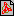StartsidanMy way of understanding ElectromagnetismMy way 2017.pdfIn this paper I show how Maxwell's equations can be derived from the assumption that there is no such thing as isolated electric or magnetic fields, but only radiations.

I give a picture of the capacitor, the inductor and charged particles based on radiations. When we skip the fields and concentrate on radiations it is easy to explain forces between particles (a force is the change in total energy to distance), even the nuclear force, and to explain where potential and kinetic energy is stored. This includes gravity as well.

The idea behind this paper started with simple measurements on transmission lines. You can follow the measurements on Youtube:

Startsidan